# Numeracy for all (VSO)

Marilyn Leask, Emma Goto, Mary Watkins, Richard Procter | View as single page | Feedback/Impact

## Teaching approaches overview

TRANSLATING CURRICULUM WORDS INTO CHILDREN’S LEARNING

PETER LACEY

Mary Watkins, a VSO specialist teacher, has produced a publication called ‘Numeracy for All –Resources for teaching mathematics’. It is described as ‘a guide for teachers and trainers on how to make and use low cost/ no cost teaching and learning aids to encourage active and playful learning for mathematics in the classroom. ‘It is wonderful!  It is intended to be used by adults who are working with young children in developing countries who may not have full access to formal schooling.  VSO workers are facilitating this work. As part of the VSO/MESH numeracy for all project, some work has been done by others to “map” the activities suggested in Mary’s publication to aspects and elements of the curriculum for young children up to the age of 7 years.

This paper attempts to help those adults working with the children on their mathematics to bridge the gap between the written words of the curriculum and the activities and outcomes of learning.  And, of course, benefit from Mary’s advice on the way.

PL August 2019

“To teach is to cause to learn”, Joseph Jacotot, Enseignement Universel, 1823

“Learning is not the product of teaching.  Learning is the product of the activity of learners”, John Holt, How Children Fail, 1964

When you look at a mathematics curriculum or syllabus it is usually a list of mathematical facts, knowledge, concepts and skills.  This list may describe what is to be taught over a given period, but more critically, by inference or design, it sets out what children should know, understand and be able to do by the end of that period.  We may call these statements “expectations”.  For the purpose of this exercise, I have chosen the expectations for a seven-year-old as described in the National Curriculum for mathematics in England.  You will find the full list in Appendix 1 at the end of this paper.  You may wish to replace this with the list from your country.  If you do this, try to start each section with “Children should be able to ......”, as in the example.

These lists do not describe the full curriculum.  They may be described as the tip of an iceberg, and, like an iceberg, there is far more of it under the water and out of sight.  See Figure 1.

Above the dotted line is normally the visible part, but the most important part lies below this dotted line.  Have this picture in front of you while you read the rest of this introduction.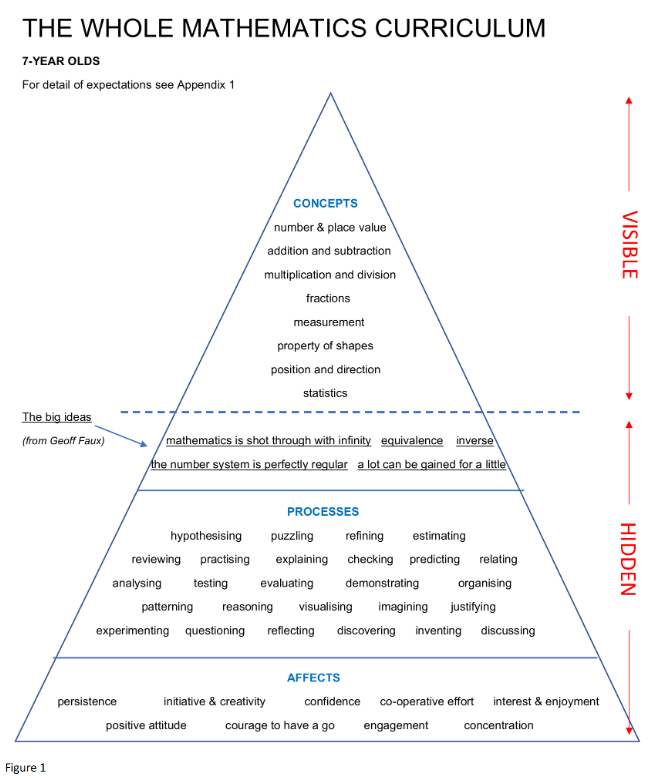The top part, above the dotted line, summarises the lists we have been looking at and referred to above. I have called this area the CONCEPT DOMAIN because it describes what the children should know and understand. A characteristic of mathematics is its interconnectivity. The curriculum lists in this concept domain do not prescribe a learning sequence. John Holt describes this well.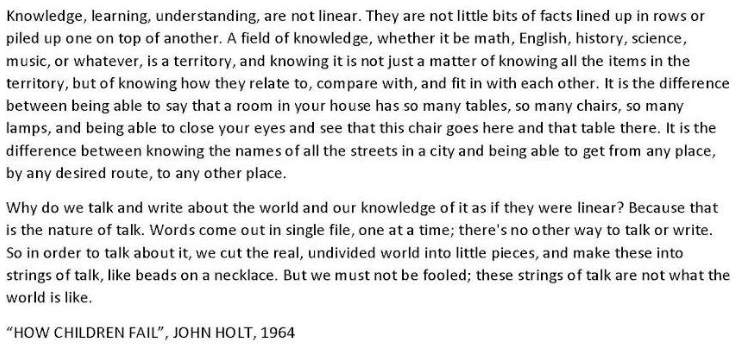By interconnectivity I mean that the “bits” of mathematics join with other “bits” to form a lattice.  If I know one mathematical fact, I can infer or deduce other facts that may be close to it on the lattice.  For example, if I know that 3 + 5 = 8, I also know that 5 +3 = 8 and that 8 = 5+3.  I may also know that 300 + 500 = 800, and so on. Click here or to see a dynamic Powerpoint picture of this particular lattice.  Scroll through the other slides in this sequence see the process which I call membering and remembering.  The last slide was my answer to a person who asked how I learned mathematics.

Recognising and constructing connected facts depends on being aware of what Geoff Faux called the big ideas of mathematics.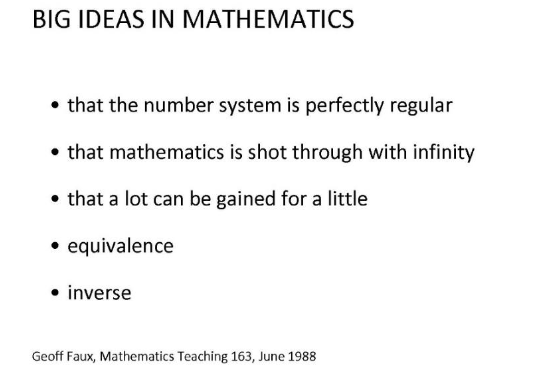These form the bedrock of the concept domain. They are the underlined concepts on your picture, and the underlying concepts of the curriculum. If children understand these big ideas, then they are able to make connections themselves. For example, the links across 5 + 3 = 8, 50 + 30 = 80 and 500000 + 30000 = 800000 are made because of the regularity of the number system and the fact that numbers extend to infinity. The link between 5 + 3 = 8 and 8 –3 = 5is made because of the concept of inverse. The statement 5 + 3 = 4 + 4 = 3 + 5 = 8 illustrates equivalence. These examples show how knowing one fact enables the knowing of other facts. Such is nature of mathematics: a lot can be gained from a little.

Giving children images of the number system helps them grasp these big ideas. Number lines, number squares and Gattegno tens charts are particularly helpful. Click here to see some examples.

Resources such as Cuisenaire rods, the spike abacus and place value cards facilitate access to and complement the use of these images. Children should be given sufficient time using these resources and images so that they can begin to “see” them in their mind. From image to imagined. It is my view that the images should be physically available throughout a child’s primary schooling. There are some suggestion on how these images may be used in my book called “Building Numeracy”.

In order to acquire mathematical knowledge, skills and understanding, children need to work mathematically. John Holt’s quote at the top of the page makes this abundantly clear. The teacher cannot learn for her students. The teacher needs to encourage children to acquire mathematical habits so that they may acquire the necessary concepts. On your picture, these are described in the PROCESS DOMAIN. Notice how all the words end in ....ing. I first wrote about the primacy of stimulating learning in the classroom in 1991, after two years of observing the teaching of mathematics, and you may wish to read the first part of the paper “Fitting the Pieces Together”. Years later I worked with teachers to develop progression pathways in the process domain and we published a paper called “A Curriculum for Learning”, which may be of interest. In this paper we argued that there are two ways of looking at progress in mathematics: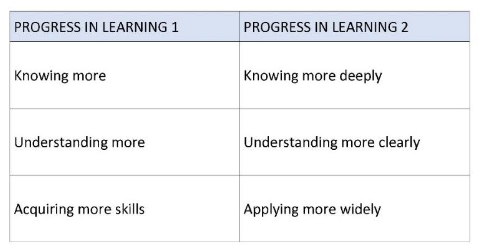One refers to knowing more, understanding more, and acquiring more skills; the other refers to knowing more deeply, understanding more clearly and applying more widely. Some call this the route to mastery.  Mathematical processes enable this deeper and more secure understanding.

Reflect on your own classroom.  How many of those mathematical processes can you see?  What can you do to encourage more of those processes to happen? Think about the tasks you give to children.  Do they encourage a range of mathematical processes?  If not, how could you alter them so they do?  For example, rather than give children a set of simple additions, ask them to find how many addition calculations give a sum of, say, 11.  Let children talk about what they have noticed and found out.  As a teacher, notice the ...ings, notice any big ideas being encountered.  As the children are learning mathematics, notice what it is they have learnt.  We call these sorts of activities “open-ended”.  Rather than define a lesson by its end-points (learning objectives), why not define it by its starting points–use a rich mathematical activity.

Visit the Association of Teachers of Mathematics (ATM) website to see their publications for example for 6 and 7-year olds, where you will find a range of rich tasks which require children to exercise mathematical processes. The NRICH website has free-to-use resources.

Share with other teachers how you have generated those mathematical processes in your classroom. Remember learning children will end up having learnt mathematics.  Talk with the other teachers about what the children have learnt. Though the process domain of the curriculum is hidden and rarely made explicit, it is my view that without children working mathematically, (as mathematicians do) secure learning is less likely.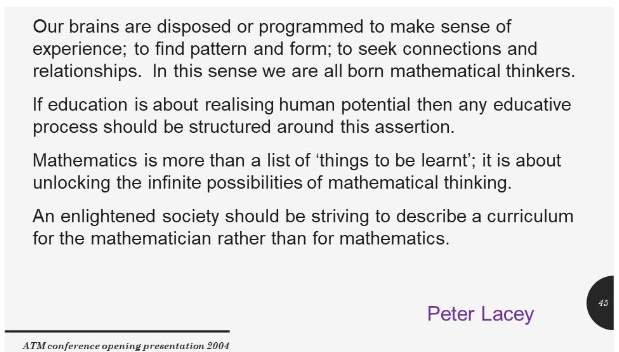And now to the deepest level of the curriculum.  You will see from your picture that along the bottom of the triangle we have what I call the AFFECT DOMAIN.  The attitudes and dispositions of learners have a significant impact on how and what they learn.  See below the John Holt quote on the effect of fear on learning.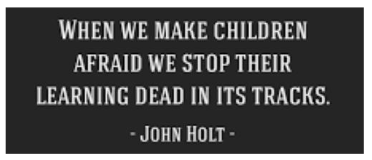How we behave as teachers has consequences for learners.  I strongly recommend that you read John Holt’s book “How Children Fail” to find out more about this.  It shaped my thinking when I started my career in teaching.  Look at the nine elements in this domain. Consider carefully how you might encourage these attitudes when working with children.  Permission to sometimes get things wrong, and to learn from mistakes, is part of this story.  Edison, the inventor of the electric light bulb, talks about the hard work and persistence needed to achieve success.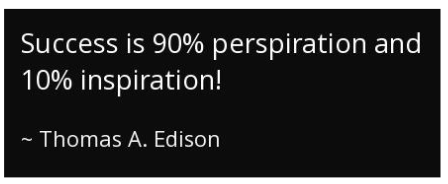Learning mathematics takes effort.  Avoiding the “hard bits” of mathematics prevents the learner developing as a mathematician and diminishes the image of what mathematics is about.  Encourage children to be creative by giving them tasks where they have to make choices.  Let them work together sometimes to share, test and evaluate their ideas.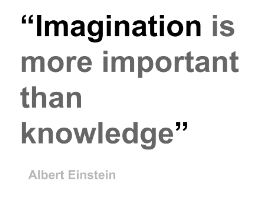I once admitted to a friend that I could not play the piano.  He replied by telling me that I might be able to play the piano, but I had chosen not to learn how to.  Many people admit to not being able to ‘do’ mathematics.

It’s our job as teachers to change that mindset and to help others choose to learn mathematics.  Think of how that may be possible.  Once the choice is made then the learning journey is under way.

APPENDIX 1

National Curriculum for England (Mathematics)

The expectations of a seven-year-old (by the end of Key Stage 1)

Number - Number and Place Value

Children should be able to:

Count in steps of 2, 3, and 5 from 0, and in tens from any number, forward and backward

Recognise the place value of each digit in a two-digit number

Identify, represent and estimate numbers using different representations, including the number line

Compare and order numbers from 0 to 100; use <, > and = signs

Read and write numbers to at least 100 in numerals and in words

Use place value and numbers facts to solve problems.

Children should be able to:

Solve problems with addition and subtraction: using concrete objects and pictorial representations, including those involving numbers, quantities and measures; applying their increasing knowledge of mental and written methods

Recall and use addition and subtraction facts to 20 fluently, and derive and use related facts up to 100

Add and subtract numbers using concrete objects, pictorial representations, and mentally, including: a two-digit number and ones; a two-digit number and tens; two two-digit numbers; adding three one-digit numbers

Show that addition of two numbers can be done in any order (commutative) and subtraction of one number from another cannot

Recognise and use the inverse relationship between addition and subtraction and use this to check calculations and solve missing number problems.

Number –Multiplication and Division

Children should be able to:

Recall and use multiplication and division facts for the 2, 5 and 10 multiplication tables, including recognising odd and even numbers

Calculate mathematical statements for multiplication and division within the multiplication tables and write them using the multiplication (×), division (÷) and equals (=) signs

Show that multiplication of two numbers can be done in any order (commutative) and division of one number by another cannot

Solve problems involving multiplication and division, using materials, arrays, repeated addition, mental methods, and multiplication and division facts, including problems in contexts.

Number –Fractions

Children should be able to:

Recognise, find, name and write fractions 1/3, 1/4, 2/4, and 3/4 of a length, shape, set of objects or quantity

Write simple fractions for example, ½ of 6 =3 and recognise the equivalence of 2/4 and ½.

Measurement

Children should be able to:

Choose and use appropriate standard units to estimate and measure length/height in any direction (m/cm); mass (Kg/g); temperature (⁰C); capacity (litres/ml) to the nearest appropriate unit, using rulers, scales, thermometers and measuring vessels

Compare and order lengths, mass, volume/capacity and record the results using >, < and = signs

Recognise and use symbols for pounds (£) and pence (p); combine amounts to make a particular value

Find different combinations of coins that equal the same amounts of money

Solve simple problems in a practical context involving addition and subtraction of money of the same unit, including giving change

Compare and sequence intervals of time

Tell and write the time to five minutes, including quarter past/to the hour and draw the hands on a clock face to show these times

Know the number of minutes in an hour and the number of hours in a day.

Geometry - Properties of Shape

Children should be able to:

Identify and describe the properties of 2-D shapes, including the number of sides and line symmetry in a vertical line

Identify and describe the properties of 3-D shapes, including the number of edges, vertices and faces

Identify 2D shapes on the surface of 3-D shapes, [for example, a circle on a cylinder and a triangle on a pyramid]

Compare and sort common 2-D and 3-D shapes and everyday objects.

Geometry - Position and Direction

Children should be able to:

Order and arrange combinations of mathematical objects in patterns and sequences

Use mathematical vocabulary to describe position, direction and movement, including movement in a straight line and distinguishing between rotation as a turn and in terms of right angles for quarter, half and three-quarter turns (clockwise and anti-clockwise).

Statistics

Children should be able to:

Interpret and construct simple pictograms, tally charts, block diagrams and simple tables

Ask and answer simple questions by counting the number of objects in each category and sorting the categories by quantity